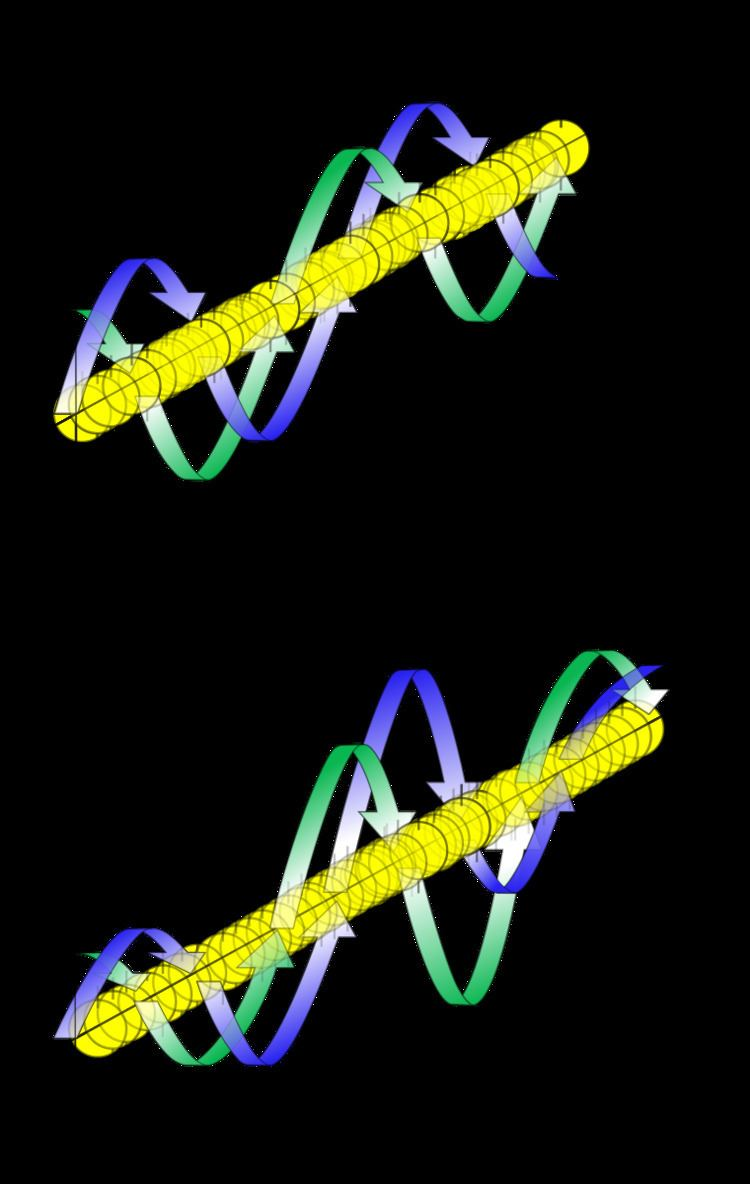# Free particle

Updated on
Covid-19In physics, a free particle is a particle that, in some sense, is not bound by an external force, or equivalently not in a region where its potential energy varies. In classical physics, this means the particle is present in a "field-free" space. In quantum mechanics, it means a region of uniform potential, usually set to zero in the region of interest since potential can be arbitrarily set to zero at any point (or surface in three dimensions) in space.

## Classical free particle

The classical free particle is characterized simply by a fixed velocity v. The momentum is given by

p = m v

and the kinetic energy (equal to total energy) by

E = 1 2 m v 2

where m is the mass of the particle and v is the vector velocity of the particle.

## Mathematical description

A free quantum particle is described by the Schrödinger equation:

2 2 m 2   ψ ( r , t ) = i t ψ ( r , t )

where ψ is the wavefunction of the particle at position r and time t. The solution for a particle with momentum p or wave vector k, at angular frequency ω or energy E, is given by the complex plane wave:

ψ ( r , t ) = A e i ( k r ω t ) = A e i ( p r E t ) /

with amplitude A. As for all quantum particles free or bound, the Heisenberg uncertainty principles

Δ p x Δ x 2 , Δ E Δ t

(similarly for the y and z directions), and the De Broglie relations:

p = k , E = ω

apply. Since the potential energy is (set to) zero, the total energy E is equal to the kinetic energy, which has the same form as in classical physics:

E = T 2 k 2 2 m = ω

## Measurement and calculations

The integral of the probability density function

ρ ( r , t ) = ψ ( r , t ) ψ ( r , t ) = | ψ ( r , t ) | 2

where * denotes complex conjugate, over all space is the probability of finding the particle in all space, which must be unity if the particle exists:

a l l s p a c e | ψ ( r , t ) | 2 d 3 r = 1

This is the normalization condition for the wave function. The wavefunction is not normalizable for a plane wave, but is for a wavepacket.

In this case, the free particle wavefunction may be represented by a superposition of free particle momentum eigenfunctions ϕ(k), the Fourier transform of the momentum space wavefunction:

ψ ( r , t ) = 1 ( 2 π ) 3 a l l p s p a c e A ( p ) e i ( p r E t ) / d 3 p = 1 ( 2 π ) 3 a l l k s p a c e A ( k ) e i ( k r ω t ) d 3 k

where the integral is over all k-space, and E = E ( p ) = p 2 2 m and ω = ω ( k ) = k 2 2 m (to ensure that the wavepacket is a solution of the free particle Schrödinger equation). Note that here we abuse notation and denote A ( p ) = A ( k ) and A ( k ) with the same symbol, when we should denote A ^ ( k ) = A ( k ) , where A is the p-space and A ^ the k-space function.

The expectation value of the momentum p for the complex plane wave is

p = ψ | i | ψ = a l l s p a c e ψ ( r , t ) ( i ) ψ ( r , t ) d 3 r = k

and for the general wavepacket it is

p = a l l s p a c e ψ ( r , t ) ( i ) ψ ( r , t ) d 3 r = a l l k s p a c e k | A ( k ) | 2 d 3 k

The expectation value of the energy E is (for both plane wave and general wave packet; here one can observe the special status of time and hence energy in quantum mechanics as opposed to space and momentum)

E = ψ | i t | ψ = a l l s p a c e ψ ( r , t ) ( i t ) ψ ( r , t ) d 3 r = ω

For the plane wave, solving for k and ω and substituting into the constraint equation yields the familiar relationship between energy and momentum for non-relativistic massive particles

E = p 2 2 m .

In general, the identity holds in the form

E = p 2 2 m

where p = |p| is the magnitude of the momentum vector.

The group velocity of the plane wave is defined as

v g = d ω d k

which turns out to be the classical velocity of the particle. The phase velocity of the plane wave is defined as

v p = ω k = E p = p 2 m = v 2

## Relativistic quantum free particle

There are a number of equations describing relativistic particles: see relativistic wave equations.

## References

Similar Topics
She Wore a Yellow Ribbon
Marla Maples
Bimal Soni
Topics

 B i Link H2
 L